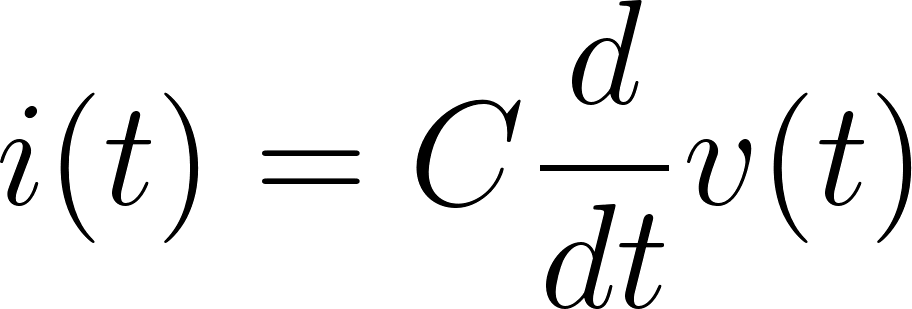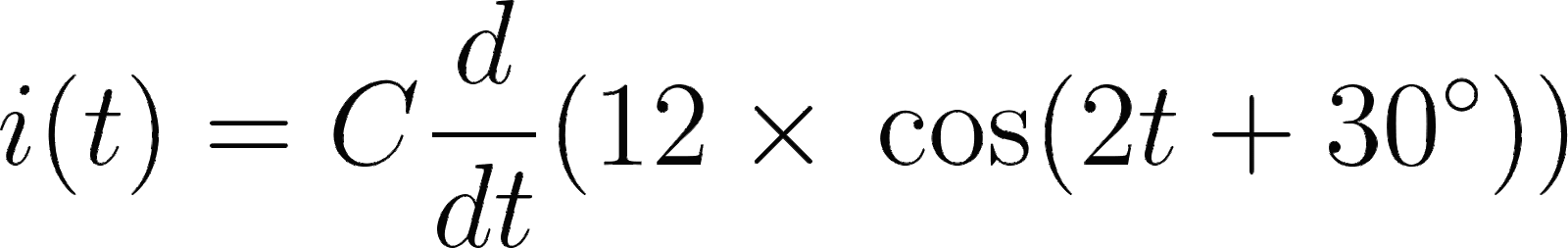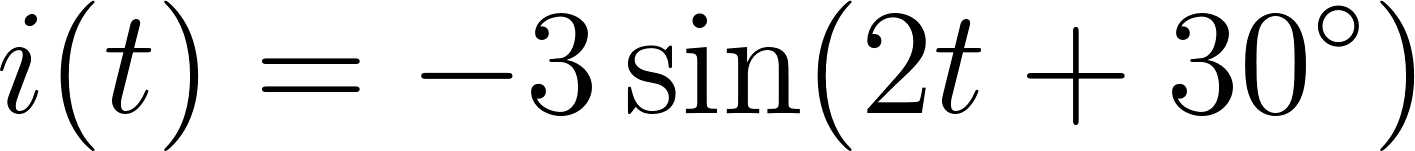Introduction To Electric Circuits - 9 Edition - Chapter 7.2 - Problem P7.2-2
Register Now

Join StudySoup

Get Full Access to Introduction To Electric Circuits - 9 Edition - Chapter 7.2 - Problem P7.2-29781118477502

# Solved: The voltage v(t) across a capacitor and current i(t) in that capacitor adhere to

Introduction to Electric Circuits | 9th Edition

Problem P7.2-2

The voltage v(t) across a capacitor and current i(t) in that capacitor adhere to the passive convention. Determine the current i(t) when the capacitance is C 0:125 F, and the voltage is v t 12 cos 2t 30 V. Hint: d dt A cos ot y A sin ot y d dt ot y Ao sin ot y Ao cos ot y p 2

Accepted Solution
Step-by-Step Solution:

Step 1 of 3

Relationship between the current and voltage of a capacitor is:###### Chapter 7.2, Problem P7.2-2 is Solved

Step 2 of 3

Step 3 of 3

Unlock Textbook Solution